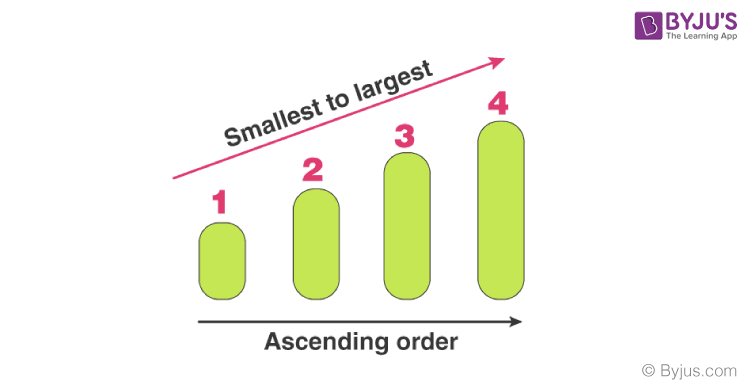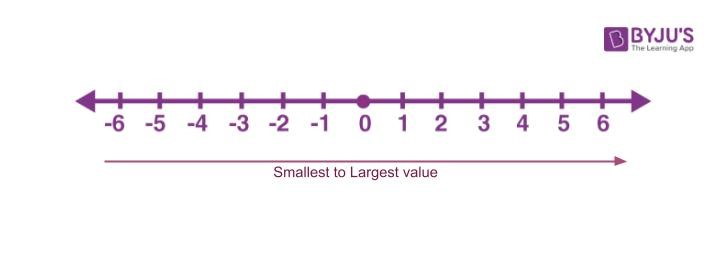# Ascending Order

Ascending order is a method of arranging numbers from smallest value to largest value. The order goes from left to right. Ascending order is also sometimes named as increasing order. For example, a set of natural numbers are in ascending order, such as 1 < 2 < 3 < 4 < 5 < 6 < 7 < 8… and so on. The less than symbol (<), is used to denote the increasing order.  The inverse method of increasing order is descending order, where the numbers are arranged in decreasing order of values.

Learn the ascending order definition/meaning, symbol/sign, examples, representation on a number line, ascending order of fractions, solved problems, etc., in this article.

## What is Ascending Order?

In Mathematics, ascending order is the process of arranging the numbers in increasing order, i.e., from smallest value to largest value. That means the numbers are arranged from left to right in increasing order. A real-life example of ascending order is an arrangement of English alphabets from A to Z.The word ‘ascending’ means going up. Hence, in the case of Mathematics, if the numbers are going up, then they are arranged in ascending order.

The other terms used for ascending order are:

• Lowest value to highest value
• Bottom value to Top value

Note: It is not necessary that the numbers are available in a pattern, to be arranged in ascending order.

## Ascending order symbol

Ascending order is denoted by the less than symbol ‘<‘. For example,

1 < 2 < 3 < 4 < 5 < 6 < 7 < 8 < 9

The above numbers are arranged in ascending order from 1 to 9.

The symbol represents that the succeeding number is greater than the preceding number in the arrangement.

The sign of ascending order represents numbers from lowest to highest, which is opposite to the concept of descending Order, where numbers are arranged from largest to smallest.

## Examples of Ascending Order

Some of the examples of numbers arranged in ascending order are given below:

• 1 <  2 < 3
• 10 < 11 < 12 < 13
• 43 < 55 < 78 < 98 < 101
• 100 < 1000 < 10000
• -10 < -9 < -8 < -7

## Ascending Order on Number Line

The concept of ascending order is been introduced in primary classes for students of Class 1. With the help of the number line, students can easily understand the arrangement of numbers in increasing order.In the above number lines numbers from -6 to 6 are arranged in ascending order. It states that numbers on the left side of 0 are smaller than the numbers on the right side of 0. As we go from left to right on the number line the value of numbers increases.

## How to Arrange Numbers in Ascending Order?

To arrange the numbers in ascending order, first, we need to compare the values and then order them in ascending order.

We can arrange here different numbers such as:

• Integers
• Negative numbers
• Fractions
• Decimals

### Arranging Integers in Ascending Order

As we know integers are numbers that can be negative, positive or zero. But they are not fractions. Let us learn how to arrange positive integers in ascending order.

• Firstly compare the number of digits in each number
• The number with lesser digits is the smallest number
• The number with the largest digits is the largest number
• If the number of digits is the same, then compare the left-most digits of the numbers
• Compare the numbers in the same manner and arrange them all from smallest to largest.
 Example: 2 < 4 < 12 < 23 < 451 < 541

### Negative Numbers in Ascending Order

Arranging negative numbers can be a little challenging for the students at the beginning. But once they have understood the logic, it will be very easy for them to arrange the numbers in ascending order.

If a bigger number is having a negative sign, then it becomes the smallest value. For example, 3 is greater than 2, but -3 is smaller than 2.

Similarly, a two-digit negative number is smaller than a single-digit negative number.

-43 < -8

 -50 < -25 < -10 < -1

### Fractions in Ascending order

There are two methods involved in finding the ascending order of the fractional numbers. Both the method will give the same solution

Method 1:

• Step 1: For a given series of fractions, first convert it into decimal numbers.
• Step 2: Find the increasing order of the decimal numbers.
• Step 3: Finally, replace the decimal values with the respective fractional numbers.

Method 2 :

• Step 1: Find the L.C.M of the denominators.
• Step 2: Divide the L.C.M value by the denominator of the fraction.
• Step 3: Multiply both the numerator and denominator of the fraction with the resultant value of step 2.
• Step 4: As a result of step 2 and step 3, compare the like fractions.
• Step 5: Since the denominators are the same, compare the numerator values of like fractions.
• Step 6: Finally, arrange the fractions in increasing order with their respective fractions given in the problem.

### Decimals in Ascending Order

To arrange the list of decimal numbers, first, check which decimal has the least whole number part. Now arrange the decimals with increasing order of whole number part.

If the whole number part is the same for two or more decimals, then arrange the decimals according to the decimal part after th decimal point, in ascending order.

Example: 1.2 < 2.3 < 3.5 < 3.6 < 3.8 < 4.3

### Ascending order of Alphabets

Same as numbers you can also arrange alphabets in ascending order and descending orders. For example : a < b < c < d < e < f < g < h < i < j <  k < l < m < n < o < p < q < r < s < t < u < v < w < x < y < z (For small alphabets).

You can reverse the order of the alphabets in the case of descending order.

## Ascending Order and Descending Order

Descending order is the contradiction of ascending order. That means it is the opposite process of writing the numbers in increasing order. Therefore, it can be mentioned as a decreasing order. In the case of descending order, for a given set of numbers, the highest valued number is written first, and the lowest valued number is written at last. It is denoted by the symbol ‘>’.

 Ascending Order Descending Order Numbers are arranged in increasing order Numbers are arranged in decreasing order Smallest to largest Largest to smallest It is represented by less than symbol: ‘<’ It is represented by greater than symbol: ‘>’ Example: 3<6<7<9<10 Example: 10>9>7>6>3

## Solved Problems on Ascending Order

Question 1: Arrange the numbers 34, 10, 6, 4, 45, 25 in ascending order.

Solution: The increasing order of the numbers are 4, 6, 10, 25, 34 and 45

Question 2: Write these numbers in ascending order: 8, 10, 92, 1, 27.

Solution: The following is the increasing order of the given numbers:

1 < 8 < 10 < 27 < 92

Question 2: Arrange the following numbers in increasing order.

63 , 72 , 42 , 26 ,34 and 102

Solution: Given , 63 , 72 , 42 , 26 ,34 and 102

63 = 6 x 6 x 6 = 216

72= 7 x 7 =49

42 = 4 x 4 = 16

26 =2 x 2 x 2 x 2 x 2 x 2 = 64

34 = 3 x 3 x 3 x 3 = 81

102 = 10 x 10 = 100

Therefore, the increasing order of the numbers are 16, 49, 64, 81, 100 and 216 and will be represented as  : 42 < 72 < 26 < 34 < 102 < 63

Question 4: Find the increasing order of the following fractions: 8/6, 2/3, 10/12 and 9/6.

Solution:

Given series: 8/6, 2/3, 10/12 and 9/6.

Step 1: Converting fractions into decimals

8/6 = 1.33

2/3 = 0.67

10/12 = 0.83

9/6 = 1.5

Step 2: Arranging the increasing order of the decimal values

0.67 < 0.83 < 1.33 < 1.5

Step 3 : Replacing the decimal values with fraction values

2/3 < 10/12 < 8/6 < 9/6

Therefore, the increasing order of the given fractions is 2/3, 10/12, 8/6 and 9/6.

Question 5: Write 3, 7, 8, 2, 10, 28, 15 in descending order.

Solution: 28 > 15 > 10 > 8 > 7 > 3 > 2 is the descending order of the given set of numbers.

## Practice Problems on Ascending Order

These problems are the worksheet for students to practice more in ascending order.

1. Arrange in ascending order: 2, 1, 7, 3, 4.
2. Write the numbers in increasing order: 90, 34, 92, 1, 35.
3. Write the numbers in ascending order using the symbol: 80, 1, 12, 10, 72.
4. Rearrange the numbers in increasing order: 18, 11, 67, 19, 07.
5. Write the numbers in increasing order: 7, 15, 90, 81, 56.

## Frequently Asked Questions on Ascending Order

Q1

### What is the ascending order and descending order?

When the numbers are written in increasing order, smallest to largest value, then it is said to be ascending, for example, 3<5<7<9<11<13 When the numbers are written in decreasing order, i.e. largest to smallest value, then it is said to be descending order. For example, 13>11>9>7>5>3
Q2

### How can we arrange the numbers in increasing order?

Suppose a set of values are given: 23, 11, 15, 9, 55, 43.
By arranging them in ascending order, we have to figure the smallest value first, which is 9.
9
Now the number greater than 9 here is 11. Therefore,
9<11
It indicates that 11 is greater than 9. In the same way, we can arrange all the numbers as;
9<11<15<23<43<55
Q3

### What is ascending order in A to Z?

Ascending order means going up from small value to high value and text from A to Z. Descending order means arranging the numbers from largest to smallest and text from Z to A.
When the names are arranged for a list, then it is usually arranged in A to Z order, alphabetically.
Q4

### How to arrange negative numbers in ascending order?

To arrange negative numbers, we should always remember that the highest number with a minus sign (-) or negative symbol, is the smallest value.For example, -3, -8, -10, -4, -9 are the integers. Then on arranging them in increasing order, we get;
-10, -9, -8, -4, -3
Hence, -10 is the smallest value here, and -3 is the largest one.
Q5

### What is the sign of ascending and descending orders?

Ascending order is represented by < (less than) symbol, whereas descending order is represented by > (greater than) symbol.
Q6

### How to arrange fractions with the same denominator in ascending order?

If the fractions have the same denominator, the fraction with the smallest numerator comes first. For example, 3/4 < 5/4 < 7/4.

Q7

### Arrange the decimals in ascending order: 0.1, -0.02, 1.2, -1.5.

The sequence 0.1, -0.02, 1.2, -1.5 arranged in ascending order is -1.5 < -0.02 < 0.1< 1.2.

Q8

### What is the ascending order of 5, 9, 18, 3, 6, 7, 12?

The ascending order of numbers 5, 9, 18, 3, 6, 7, 12 is:
3 < 5 < 6 < 7 < 9 < 12 < 18

Q9

### Does the smallest number come first in ascending order.

Yes, the smallest number comes first in ascending order.

Quiz on Ascending Order